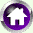#Utility, Substitution and Demand

### 9: Questions to think about

• What happens to our calculations if we change the units in which we measure utility?
• What happens to the maximum total utility when the price of a good increases? What does common sense say? Can you confirm this using the interactive exercises?
• Why did we assume diminishing marginal utility? What happens to the optimal proportion of crisps or of nachos if we do not make that assumption?
• We used the same utility function for crisps and for nachos. What happens if we alter this assumption, so that nachos have 1.1 times the utility of an equivalent number of packs of crisps?